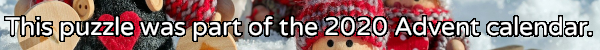mscroggs.co.uk
mscroggs.co.uksubscribe

## 25 DecemberIt's nearly Christmas and something terrible has happened: you've just landed in a town in the Arctic circle with a massive bag of letters for Santa, but you've lost to instructions for how to get to Santa's house near the north pole. You need to work out where he lives and deliver the letters to him before Christmas is ruined for everyone.
Due to magnetic compasses being hard to use near the north pole, you brought with you a special Advent compass. This compass has nine numbered directions. Santa has given the residents of the town clues about a sequence of directions that will lead to his house; but in order to keep his location secret from present thieves, he gave each resident two clues: one clue is true, and one clue is false.
Here are the clues:
9
"The 4th digit is 4."
"The 8th digit is 4."
4
"The 3rd digit is 1."
"The 4th digit is 7."
10
"The 11th digit is a factor of 888."
"The 11th digit is a factor of 88."
13
"The 3rd digit is 1."
"The 4th digit is 6."
23
"The 10th digit is 4."
"The 10th digit is 3."
1
"The last digit is 1, 9 or 5."
"The last digit is 1."
5
"The 3rd digit is 1."
"The 4th digit is 3."
16
"The 5th digit is 3."
"The 6th digit is 3."
11
"The sequence's length is 2+1+6."
"The sequence's length is 2×1×6."
12
"The 6th to 8th digits are 3, 3, 4."
"The 7th to 9th digits are 3, 3, 4."
2
"The 7th digit is 4."
"The 8th digit is 4."
21
"The 5th digit is 3."
"The 9th digit is 9."
22
"The 9th digit is 9."
"The 10th digit is 4."
17
"The 3rd digit is 1."
"The 4th digit is 9."
15
"The 2nd* digit is 7."
"The 1st digit is 8."
14
"The 1st digit is 7."
"The 2nd digit is 7."
7
"The last digit is not 5."
"The 4th digit is 5."
3
"The 11th digit is a factor of 10."
"The 11th digit is a factor of 321."
6
"The sequence's length is 1+9+2."
"The sequence's length is 1×9×2."
20
"The 5th digit is 3."
"The 5th digit is 4."
19
"5 does not appear."
"5 appears exactly once."
24
"The 3rd digit is 1."
"The 4th digit is 8."
25
18
"The 1st digit is 3, 7 or 8."
"The sequence's length is prime."
8
"The 3rd digit is 1."
"The 4th digit is 2."
You can view the map here.

## Archive

Show me a random puzzle
▼ show ▼